﻿ 基于Meta-US-SBM模型的长株潭生态效率测度与评价 Measurement and Evaluation of Ecological Efficiency of Changsha, Zhuzhou and Xiangtan Based on Meta-US-SBM Model

Statistics and Application
Vol. 07  No. 06 ( 2018 ), Article ID: 27889 , 13 pages
10.12677/SA.2018.76067

Measurement and Evaluation of Ecological Efficiency of Changsha, Zhuzhou and Xiangtan Based on Meta-US-SBM Model

Yuhui Xie, Yulin Zhu

Central South University of Forestry and Technology, Changsha HunanReceived: Nov. 15th, 2018; accepted: Nov. 30th, 2018; published: Dec. 7th, 2018ABSTRACT

Developing a green economy is a necessary channel for Changsha, Zhuzhou and Xiangtan to achieve a two-type society. The measurement and evaluation of ecological efficiency is a suitable method for the assessment of green economy. This paper takes the “3 + 5” urban agglomeration of Changsha, Zhuzhou and Xiangtan as the research object, establishes a total factor production framework, and integrates economic efficiency, energy efficiency, environmental efficiency and ecological efficiency into a unified model, and uses the Meta-US-SBM model to measure the cities’ Eco-efficiency and its sub-efficiency in 2006-2016, and then analyze the causes of eco-efficiency based on the results of each city's efficiency. The empirical results show that the overall eco-efficiency of Changsha, Zhuzhou and Xiangtan urban agglomerations is on the rise, but Changsha is in a clear leading position, and the gap with other cities is obvious. The Changsha, Zhuzhou and Xiangtan urban agglomerations have high economic efficiency, the overall economic development is good, and the trend is stable. Under the environmental efficiency, the cities of Changsha, Zhuzhou and Xiangtan have an upward trend, but they are relatively flat compared with economic efficiency. In terms of energy efficiency, the overall Lower, needs to be treated with emphasis.

Keywords:CZT Urban Agglomerations, Meta-US-SBM Model, Eco-Efficiency1. 引言

2. 文献综述

3. 研究方法及数据说明

3.1. 研究方法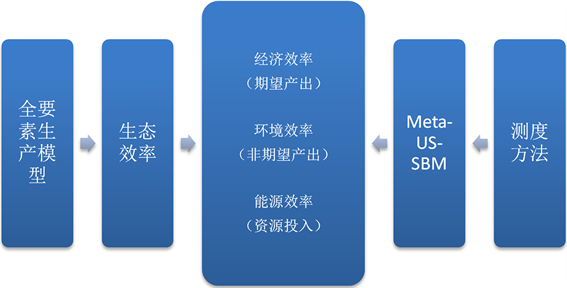Figure 1. Research ideas

3.1.1. Meta-US-SBM模型

DEA模型最初由Charnes、Cooper和Rhodes  提出，具有较多优点，例如，无须假设生产函数形式，不受指标量纲影响，不需要估计参数，可同时考虑多投入多产出的情形，实现了客观赋权，可以进行效率分解，还可以考虑环境污染这类坏产出，因此被广泛用于投入产出的评价。随着问题的逐步拓展与深入，研究发现DEA方法测度绿色效率时仍然存在一些重要而关键的问题。Huang根据最新文献研究进展，给出了更具综合性的测度模型——Meta-US-SBM模型：

$\begin{array}{l}{P}^{meta}=\left\{\left(x,y,b\right):{\sum }_{h=1}^{H}{\sum }_{n=1}^{{N}_{h}}{\xi }_{n}^{h}{x}_{n}^{h}\le {x}^{h};{\sum }_{h=1}^{H}{\sum }_{n=1}^{{N}_{h}}{\xi }_{n}^{h}{y}_{n}^{h}\le {y}^{h};\\ \text{\hspace{0.17em}}\text{\hspace{0.17em}}\text{\hspace{0.17em}}\text{\hspace{0.17em}}\text{\hspace{0.17em}}\text{\hspace{0.17em}}\text{\hspace{0.17em}}\text{\hspace{0.17em}}\text{\hspace{0.17em}}\text{\hspace{0.17em}}\text{\hspace{0.17em}}{\sum }_{h=1}^{H}{\sum }_{n=1}^{{N}_{h}}{\xi }_{n}^{h}{b}_{n}^{h}\le {b}^{h};{\xi }_{n}^{h}\ge 0;n=1,2,\cdots ,{N}_{h};h=1,2,\cdots ,H\right\}\end{array}$ (式1)

$\left[\text{Meta-US-SBM}\right]{\rho }_{ko}^{Meta*}=\mathrm{min}\frac{1+\frac{1}{M}\underset{m=1}{\overset{M}{\sum }}\frac{{s}_{mko}^{x}}{{x}_{mko}}}{1-\frac{1}{R+J}\left(\underset{r=1}{\overset{T}{\sum }}\frac{{s}_{rko}^{y}}{{y}_{rko}}+\underset{j=1}{\overset{J}{\sum }}\frac{{s}_{jko}^{b}}{{b}_{rko}}\right)}$

$s.t.\text{\hspace{0.17em}}\text{\hspace{0.17em}}{x}_{mko}-\underset{h=1}{\overset{H}{\sum }}\underset{n=1,\ne 0\text{\hspace{0.17em}}\text{if}\text{\hspace{0.17em}}h=k}{\overset{{N}_{h}}{\sum }}{\xi }_{n}^{h}{x}_{mhn}+{s}_{mko}^{x}\ge 0$

$\underset{h=1}{\overset{H}{\sum }}\underset{n=1,\ne 0\text{\hspace{0.17em}}\text{if}\text{\hspace{0.17em}}h=k}{\overset{{N}_{h}}{\sum }}{\xi }_{n}^{h}{y}_{rhn}-{y}_{rko}+{s}_{mko}^{x}\ge 0$

${b}_{jko}-\underset{h=1}{\overset{H}{\sum }}\underset{n=1,\ne 0\text{\hspace{0.17em}}\text{if}\text{\hspace{0.17em}}h=k}{\overset{{N}_{h}}{\sum }}{\xi }_{n}^{h}{b}_{jhn}+{s}_{jko}^{b}\ge 0$

$1-\frac{1}{R+J}\left(\underset{r=1}{\overset{R}{\sum }}\frac{{s}_{rko}^{y}}{{y}_{rko}}+\underset{j=1}{\overset{J}{\sum }}\frac{{s}_{jko}^{b}}{{b}_{jko}}\right)\ge \epsilon$

${\xi }_{n}^{h},{s}^{x},{s}^{y},{s}^{b}\ge 0$

$m=1,2,\cdots ,M;r=1,2,\cdots R;j=1,2,\cdots ,J$ (式2)

Meta-US-SBM的评价思路如下：

1、先根据异质性特征将样本分为若干群组，群组内部各决策单元具有相同或高度相近的技术水平，每个群组分别构建生产技术前沿(称为“群组前沿”)由此可以测算得到群组效率，进一步再给予个群组前沿构建一个包络所有族群生产可能性集的共同生产可能性集，即所有群组的共同前沿面(见图2)，称为“共同前沿”；2、采用非径向处理好松弛变量，并运用超效率模型概念处理好更高的数据只能落在生产沿面的问题；3、使用非导向处理使得可以同时从投入和产出两个方面进行测量；4、利用全局参比技术，对面板数据进行处理，以解决跨期可比性和可传递性问题。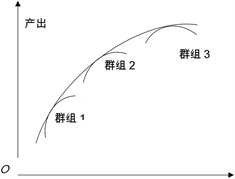Figure 2. Common Frontier Model

3.1.2. 能源效率的测度

${\rho }_{ko}^{Meta*}=\mathrm{min}\left(1+\frac{1}{M}\underset{m=1}{\overset{M}{\sum }}\frac{{s}_{mko}^{x}}{{x}_{mko}}\right)$

$s.t.\text{\hspace{0.17em}}\text{ }{x}_{mko}-\underset{h=1}{\overset{H}{\sum }}\underset{n=1,\ne 0\text{\hspace{0.17em}}\text{if}\text{\hspace{0.17em}}h=k}{\overset{{N}_{h}}{\sum }}{\xi }_{n}^{h}{x}_{mhn}+{s}_{mko}^{x}\ge 0$

$\underset{h=1}{\overset{H}{\sum }}\underset{n=1,\ne 0\text{\hspace{0.17em}}\text{if}\text{\hspace{0.17em}}h=k}{\overset{{N}_{h}}{\sum }}{\xi }_{n}^{h}{y}_{rhn}-{y}_{rko}\ge 0$

${b}_{jko}-\underset{h=1}{\overset{H}{\sum }}\underset{n=1,\ne 0\text{\hspace{0.17em}}\text{if}\text{\hspace{0.17em}}h=k}{\overset{{N}_{h}}{\sum }}{\xi }_{n}^{h}{b}_{jhn}\ge 0$

${\xi }_{n}^{h},{s}_{mko}^{x}\ge 0$

$m=1,2,\cdots ,M;r=1,2,\cdots R;j=1,2,\cdots ,J$ (式3)

$E{E}^{Meta}=\frac{{x}_{e}-{s}_{e}^{Meta}}{{x}_{e}}$

3.1.3. 经济效率的测度

${\rho }_{ko}^{Meta*}=\mathrm{min}\frac{1}{1-\frac{1}{R}{\sum }_{r=1}^{R}\frac{{s}_{rko}^{y}}{{y}_{rko}}}$

$s.t.\text{\hspace{0.17em}}\text{ }{x}_{mko}-\underset{h=1}{\overset{H}{\sum }}\underset{n=1,\ne 0\text{\hspace{0.17em}}\text{if}\text{\hspace{0.17em}}h=k}{\overset{{N}_{h}}{\sum }}{\xi }_{n}^{h}{x}_{mhn}\ge 0$

$\underset{h=1}{\overset{H}{\sum }}\underset{n=1,\ne 0\text{\hspace{0.17em}}\text{if}\text{\hspace{0.17em}}h=k}{\overset{{N}_{h}}{\sum }}{\xi }_{n}^{h}{y}_{rhn}-{y}_{rko}+{s}_{rko}^{x}\ge 0$

${b}_{jko}-\underset{h=1}{\overset{H}{\sum }}\underset{n=1,\ne 0\text{\hspace{0.17em}}\text{if}\text{\hspace{0.17em}}h=k}{\overset{{N}_{h}}{\sum }}{\xi }_{n}^{h}{b}_{jhn}=0$

${\xi }_{n}^{h},{s}_{jko}^{b}\ge 0;m=1,2,\cdots ,M;$

$r=1,2,\cdots R;j=1,2,\cdots ,J$ (式4)

3.1.4. 环境效率的测度

${\rho }_{ko}^{Meta*}=\mathrm{min}\frac{1}{1-\frac{1}{J}{\sum }_{j=1}^{J}\frac{{s}_{jko}^{b}}{{b}_{jko}}}$

$s.t.\text{\hspace{0.17em}}\text{ }{x}_{mko}-\underset{h=1}{\overset{H}{\sum }}\underset{n=1,\ne 0\text{\hspace{0.17em}}\text{if}\text{\hspace{0.17em}}h=k}{\overset{{N}_{h}}{\sum }}{\xi }_{n}^{h}{x}_{mhn}\ge 0$

$\underset{h=1}{\overset{H}{\sum }}\underset{n=1,\ne 0\text{\hspace{0.17em}}\text{if}\text{\hspace{0.17em}}h=k}{\overset{{N}_{h}}{\sum }}{\xi }_{n}^{h}{y}_{rhn}-{y}_{rko}=0$

${b}_{jko}-\underset{h=1}{\overset{H}{\sum }}\underset{n=1,\ne 0\text{\hspace{0.17em}}\text{if}\text{\hspace{0.17em}}h=k}{\overset{{N}_{h}}{\sum }}{\xi }_{n}^{h}{b}_{jhn}+{s}_{jko}^{b}\ge 0$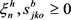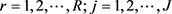(式5)

3.2. 研究指标及数据选取

1) “好”产出(期望产出)。选用各地区的实际地区生产总值(GDP)，换算为2007年不变价。

2) “坏”产出(非期望产出)。现代社会环境污染物颇多，相互之间既有差异有存在较高程度的相关性，且具有奇异值。现有文献主要考虑了SO2和CO2这两类污染物(如Li，2012)，本文根据数据可得性，选用了6个指标：CO2排放量、SO2排放量、废水排放总量、废水中化学需要量(COD)、废水中氨氮排放量和烟(粉)尘排放量。为避免高相关性和奇异值的影响，借鉴Huang (2014)利用熵权法构建环境污染指数(Environment index, EI)，以之作为坏产出指标综合反映环境约束。其取值标准化到0~100，越大(小)意味着污染物越多(少)。

3) 资本投入。估算资本投入最常见的方法是“永续存盘法”，简要说明如下：1、当期投资指标选择。参考张军等(2004)  ，本文选取固定资本形成总额It作为当年投资。2、基期资本存量的测算。借鉴Harberger (1978)，用式5测算：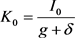(式5)(式6)

4) 劳动投入。根据可得性，采用各市历年来从业人数作为劳动投入指标。

5) 土地投入。尽管各市行政区域面积已固定，但实际利用面积和利用方式却在不断变化。根据数据可得性，本文借鉴Huang (2014)，采用各市建成区面积作为土地投入指标。

6) 水资源投入。选取供水总量为代理变量，该数据涵盖了农业与工业生产、生活和生态用水等信息。

7) 能源投入。采用各市所有能源的消费量(换算为标准煤)指标。

4. 实证结果分析

4.1. 生态效率的区域特征Table 1. Eco-efficiency value of ChangZhuTan city agglomeration according to Meta-US-SBM

1) 11年间长株潭城市群的生态效率平均水平先下降并维持在了一定水平之后，又继续上升。2016年的效率水平高于观察初期(2006年)，2009年为相对低位。由此可见，尽管受到了2008年美国金融危机影响，整体经济放缓，生态效率总体上仍然保持上升态势，说明长株潭城市群总体上正在向绿色发展转变。

2) 如图3，生态效率较高的为长沙和常德，其他城市的生态效率明显较低，而较低的6所城市又可分为中、低两档，长株潭呈现“三段式”现象。具体地，长沙和常德一直保持在较高的生态效率水平，其中长沙最为显著，2006年为最低水平，之后快速上升并远远超过其他区域，也远远高于常德和全国平均水平。而常德的平均效率值大于1，且方差较小，与其他中低地区相比，效率值较高且稳定。根据效率平均值的相对比较，湘潭、益阳和娄底为生态效率中等地区(Mean = 0.75 − 0.85)，湘潭的平均效率值处于中等(0.754)，但是生态效率整体呈现下降趋势。益阳的生态效率波动较小，趋向于稳定。娄底与益阳的生态效率相比，平均效率虽然接近，但是其波动更大，且大部分时间处于下降趋势。相对低生态效率的地区为株洲、岳阳、衡阳(Mean = 0.69 − 0.75)。株洲的生态效率为最低(0.575)，但是其自2009年开始一直呈现上涨趋势，且近几年增长强劲。岳阳生态效率整体平稳，一直处于中下水准，但是逐年上升。衡阳与岳阳的生态效率趋势整体相近，但其增长速率低于岳阳，在观测初期生态效率大于岳阳的情况下，观测末期与平均效率均低于岳阳。

3) 各城市的生态效率跨期变化不大，路径依赖较为严重。其中6所城市的平均增长率均小于0.02，只有湘潭与株洲的平均增长率大于0.02，其中株洲的平均变化率更是达到了0.0901。另外，湘潭、益阳和娄底的平均效率负，且湘潭的负增长率大于了绝大多数城市的增长率，而益阳与娄底的负增长率业与其他大多数城市的增长率相近。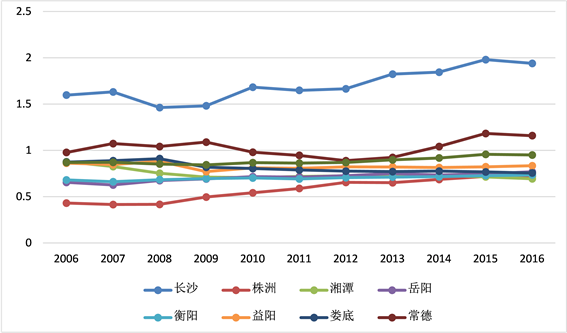Figure 3. Eco-efficiency value of ChangZhuTan city agglomeration according to Meta-US-SBM

4.2. 子效率的区域特征

4.2.1. 经济效率的区域特征Table 2. Economic-efficiency value of ChangZhuTan city agglomeration according to Meta-US-SBM

1) 总体上，经济效率分为3个档次。长沙的经济效率较高，而岳阳、常德、衡阳、株洲4市的经济效率次之，湘潭、益阳、娄底3市的区域经济效率相对较低。

2) 如图4，动态比较，长沙在观察期间(2006~2016)保持了很高的经济效率且效率有明显提升(平均增长率大于0.01)，但湘潭、益阳、娄底3市的经济效率不仅相对较低，而且增长缓慢(平均增长率小于0.01)，甚至呈现下降态势。另外，岳阳、常德、衡阳、株洲4市的经济效率相对较高，除岳阳外，平均效率均大于0.01，上升趋势显著。

3) 各市的经济效率增长趋势平稳，但在2008~2009之间受到了一定的限制，可能是由于金融危机的影响，而大部分城市的经济效率增速在之后又有了回升态势。

4.2.2. 环境效率的区域特征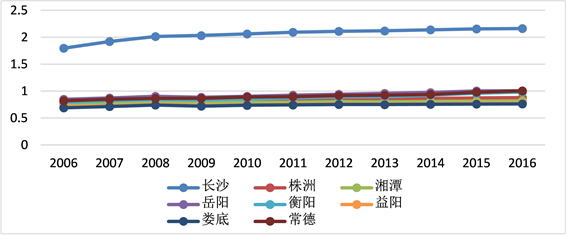Figure 4. Economic-efficiency value of ChangZhuTan city agglomeration according to Meta-US-SBMTable 3. Economic-efficiency value of ChangZhuTan city agglomeration according to Meta-US-SBM

1) 总体上，环境效率长沙、常德的环境效率较高(平均效率大于0.8)，益阳、娄底、湘潭的环境效率次之(平均效率大于0.65)，岳阳、衡阳、株洲的环境效率相对最低。

2) 如图5动态比较，长沙、常德、娄底在观察期间(2006~2016年)保持了较高的环境效率，但平均增长率均较低(小于0.01)，而益阳的环境效率虽有起伏，但是环境效率较高，且有着较高的平均增长率(大于0.02)。另外株洲、岳阳的环境效率虽然相对较低，但是平均增长率较高(大于0.025)，而湘潭、衡阳的环境效率相对较低，且平均增长率较低(小于0.01)。

3) 由历年的平均环境效率可知，各市的环境效率整体增长平稳且较为缓慢，2008年的美国金融危机、欧洲债务危机对环境效率的影响不明显，但是2011年的平均环境效率迎来一个峰值，其原因可能是十二五大力提倡的绿色发展，对于长株潭城市群建立资源节约型、环境友好型社会起到了良好的政策导向作用。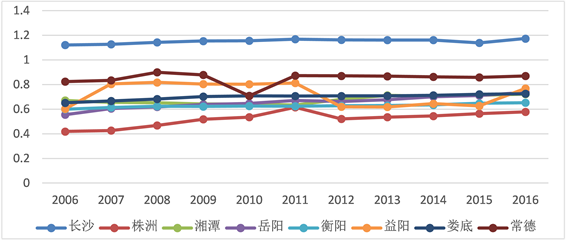Figure 5. Environmental-efficiency value of ChangZhuTan city agglomeration according to Meta-US-SBM

4.2.3. 能源效率的区域特征Table 4. Energy-efficiency value of ChangZhuTan city agglomeration according to Meta-US-SBM

1) 总体上看，长株潭城市群的能源效率较低，平均效率仅为0.623，且没有平均效率大于1的城市。而其中，长沙、株洲的能源效率较高，而常德、岳阳、衡阳次之，湘潭、益阳、娄底的能源效率相对最低。

2) 动态比较，大部分城市的能源效率平均增长率为负，且岳阳的平均增长率甚至达到了−0.03，而仅为正向增长的株洲、湘潭，增长率也较低(小于0.01)，这说明了长株潭城市群的整体能源效率是在下降的，资源浪费和能源耗散是极为严重的发展短板。

3) 如图6，长株潭城市群能源效率从2006年一直下降到2012年然后趋于平稳，受金融危机、环境政策的影响并不明显。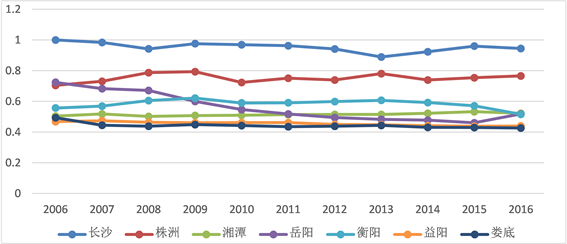Figure 6. Energy-efficiency value of ChangZhuTan city agglomeration according to Meta-US-SBM

5. 结论与讨论

1) 自2006年以来长株潭城市群生态效率在观测期间整体上升，这说明其发展模式呈现出优化趋势。其中长沙的领先地位显著，且具有不断拉开差距的态势。长沙作为城市群的核心，具有的优势与现实相符，但其与其他城市越来越大的差距也意味着各城市的锁定效应明显，追赶效应较弱。各市的生态效率路径依赖较为明显，呈现出梯度收敛现象，难以出现跨阶段的发展。因此，各市需要找准自己的薄弱环节，因地制宜地采取策略发展好其绿色经济。而长沙在保证自己发展的同时，需要发挥其核心作用，扩大其空间溢出效应，带动其他城市的绿色发展。

2) 长株潭城市群的各市子效率显示的特征各有不同，也意味着各城市的优先发展模式不同，需要重点考虑的短板也不同。在能源效率方面，虽然长沙、株洲等城市相对较高，但与经济效率和环境效率相比较，都处于明显劣势，各市均需要重点对待，在注重资源开发的同时，需要提高资源利用效率问题，大力发展科技创新、引进人才等政策是可行的方法。另外需要严格控制企业，尤其是大型工业企业的能源使用行为，避免在资源丰富环境下的浪费行为。

3) 从长株潭城市群的经济效率来看，长株潭城市群经济发展整体良好，但2008年金融危机对各市影响显著，使其有着明显的负效应，但是在此之后各市恢复良好且趋势稳定。各城市需要保持好上升态势以靠近长沙的发展速度，形成追赶效应来促进整体发展。再者，为了能够更好地发展绿色经济，各市需要坚持以供给侧结构性改革为主线，大力实施创新，引领开放崛起战略，并且不断优化产业结构，以打造健康、可持续的城市群生态环境，促进各地区经济、资源和环境的协调发展。

4) 在环境效率下，长株潭城市群各市均具有上升趋势，但与经济效率相比相对平缓。各市应该效仿长沙、常德，积极建立环保意识、做好城区规划、大力发展循环经济，并对污染企业进行适当关停以及整体改良。株洲的环境效率虽然落后但近年来一系列改革使得上升显著，这也说明了株洲政府的积极政策效果明显，各市政府需要学习株洲，积极开展环保工作，为优质的绿色发展助力。

5) 总体上，本文建议应注重各区域和资源、环境、经济各子系统的协调发展；整合、优化资源配置，提高趋于技术创新能力和增强人们的环保意识，培养和凝聚人力资本；以协调、可持续发展为理念，整合资源、保护环境，实现资源、环境和经济的协同发展。

Measurement and Evaluation of Ecological Efficiency of Changsha, Zhuzhou and Xiangtan Based on Meta-US-SBM Model[J]. 统计学与应用, 2018, 07(06): 580-592. https://doi.org/10.12677/SA.2018.76067

1. 1. Huang, J., Yang, X., Cheng, G., et al. (2014) A Comprehensive Eco-Efficiency Model and Dynamics of Regional Eco-Efficiency in China. Journal of Cleaner Production, 67, 228-238. https://doi.org/10.1016/j.jclepro.2013.12.003

2. 2. Schaltegger, S. and Sturm, A. (1990) Ecological Rationality. Die unternehmung, 44, 273-290.

3. 3. Seppala, J., Melanen, M. and Maenpaa, I. (2005) How Can the Eco-Efficiency of a Region Be Measured and Monitored? Journal of Industrial Ecology, 9, 117-130. https://doi.org/10.1162/108819805775247972

4. 4. 李丽萍, 田春秀, 国冬梅. 生态效率——OECD全新环境管理经验[J]. 环境与可持续发展, 2000(1): 33-36.

5. 5. 周国梅, 彭昊, 曹凤中. 循环经济和工业生态效率指标体系[J]. 城市环境与城市生态, 2003(6): 201-203.

6. 6. 诸大建, 朱远. 从生态效率的角度深入认识循环经济[J]. 中国发展, 2005(1): 6-11.

7. 7. 吕彬, 杨建新. 生态效率方法研究进展与应用[J]. 生态学报, 2006(11): 3898-3906.

8. 8. 张炳, 毕军, 黄和平, 等. 基于DEA的企业生态效率评价:以杭州湾精细化工业园区企业为例[J]. 系统工程理论与实践, 2008, 4(4): 159-166.

9. 9. 陈晓红, 陈石. 企业生态效率差异及技术进步贡献——基于要素密集度视角的分位数回归分析[J]. 清华大学学报:(哲学社会科学版), 2013(3): 148-157.

10. 10. 商华, 武春友. 基于生态效率的生态工业园评价方法研究[J]. 大连理工大学学报(社会科学版), 2007, 28(2): 25-29.

11. 11. 武春友, 孙源远. 基于生态承载力的工业园生态效率评价研究[J]. 管理学报, 2009, 6(6): 751-754.

12. 12. 王飞儿, 史铁锤. 基于物质代谢的中国纺织业生态效率评价[J]. 中国人口•资源与环境, 2008, 18(6): 122-126.

13. 13. 毛建素, 曾润, 杜艳春, 等. 中国工业行业的生态效率[J]. 环境科学, 2010, 31(11): 2788-2794.

14. 14. 陈傲. 中国区域生态效率评价及影响因素实证分析——以2000年-2016年省级数据为例[J]. 中国管理科学, 2008(s1): 566-570.

15. 15. 罗能生, 李佳佳. 中国城镇化进程与区域生态效率关系的实证研究[J]. 中国人口•资源与环境, 2013, 23(11): 53-60.

16. 16. 李胜兰, 初善冰, 申晨. 地方政府竞争,环境规制与区域生态效率[J]. 世界经济, 2014(4): 88-110.

17. 17. 成金华, 孙琼, 郭明晶. 中国生态效率的区域差异及动态演化研究[J]. 中国人口•资源与环境, 2014(1).

18. 18. 李佳佳, 罗能生. 城市规模对生态效率的影响及区域差异分析[J]. 中国人口•资源与环境, 2016, 26(2): 129-136.

19. 19. 付丽娜, 陈晓红, 冷智花. 基于超效率模型的城市群生态效率研究——以长株潭“3 + 5”城市群为例[J]. 中国人口•资源与环境, 2013, 23(4): 169-175.

20. 20. 李海东, 王善勇. “两型”社会建设中生态效率评价及影响因素实证分析—以2006-2009年省级面板数据为例[J]. 电子科技大学学报(社会科学版), 2012(6): 72-77.

21. 21. 张军, 吴贵英, 张吉鹏. 中国省际物质资本存量估算: 1952-2000 [J]. 经济研究, 2004(10): 35-44.

22. 22. 吴延瑞. 生产率对中国经济增长的贡献: 新的估计[J]. 经济学(季刊), 2008(3): 827-842.